Home > CCA2 > Chapter 10 > Lesson 10.3.1 > Problem10-163

10-163.
1. A small rocket is launched from five meters below ground level and reaches a height of 3 meters above the ground after 4 seconds. On the way down it is 3 meters above the ground after a total of 8 seconds. Homework Help ✎

1. What are three data points?

2. Draw a rough sketch of the height of the rocket over time.

3. Find the equation of the parabola based on the data.

4. When will the rocket hit the ground?

5. What is the domain for this function?

6. For what part of the domain is the rocket below ground?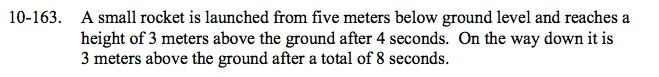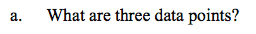Each point is (time, height). The first one is (0, −5).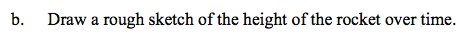Plot the three points you listed in part (a). Then sketch a parabola through them.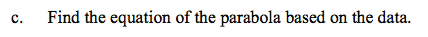See the hints and help for problem 10-154.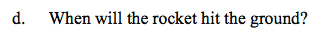Using your equation from part (c), let h (or y) = 0 and solve for t (or x).
Note: You should get two answers. Which one is it?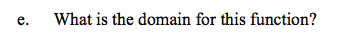Look at your graph and the answer to part (d). 0 ≤ t ≤ ?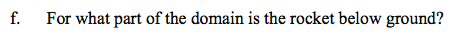Look at your graph and the answer in part (d) that you did not use. 0 ≤ t ≤ ?